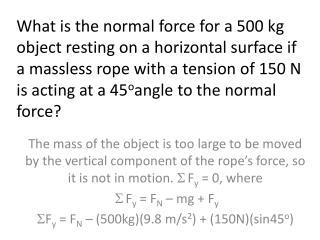DownloadDownload PresentationFree Body Diagrams

Free Body Diagrams

Download PresentationFree Body Diagrams

- - - - - - - - - - - - - - - - - - - - - - - - - - - E N D - - - - - - - - - - - - - - - - - - - - - - - - - - -
Presentation Transcript

1. What is the normal force for a 500 kg object resting on a horizontal surface if a massless rope with a tension of 150 N is acting at a 45oangle to the normal force? The mass of the object is too large to be moved by the vertical component of the rope’s force, so it is not in motion. Fy = 0, where Fy = FN – mg + Fy Fy = FN – (500kg)(9.8 m/s2) + (150N)(sin45o)

2. Free Body Diagrams • A mass at the origin is pulled by four perpendicular ropes • Find the magnitude of the resultant force. • Find the angle at which the force is acting. Now each of the ropes is pulled at 30o counterclockwise from the given axes. 3. Draw a free-body diagram and find the horizontal and vertical components of the new resultant force. 4. At what angle is the resultant force acting with respect to the x-axis?

3. Newton’s First Law • An object at rest will remain at rest and an object in motion will keep moving with constant velocity if the net force on the object is zero. • Often called the law of inertia. Inertia is the natural tendency of an object to resist a change in motion, measured quantitatively by its mass. • What happens if the net force is not zero?

4. Newton’s Second Law • Acceleration depends on the net force and on the mass of the object • Newton’s law is a vector equation. • Acceleration is in the direction of the net force, not necessarily the direction of the velocity. • Include all forces acting on an object to determine the net force. • Do not include balanced forces (such as weight and normal force) because they cancel each other

5. Newton’s Second Law of Motion The units of force are called newtons. 1 N = 1 kg . m/s2.

6. Example 4.2 • A 5.0 N horizontal force pulls a 20 kg box on a horizontal surface. A 3.0N friction force slows the motion. What is the acceleration of the object? 1. draw a free-body diagram of the box 2. write the given information 3. Apply the equation F = ma to solve the problem

7. Example 4.3 • Find the magnitude of the weight of a 3.50 kg object on the surface of the earth where w = mg

8. Newton’s Second Law of Motion Newton’s second law applies separately to each component of the force.

9. Newton’s Third Law • If object 1 exerts force on object 2, then object 2 exerts an equal and opposite force on object 1. • The two forces are acting on two different objects, therefore even though the two forces are equal and opposite, they do not necessarily cancel each other. • Often called the law of action and reaction.

10. 4.4 Newton’s Third Law of Motion For every force (action), there is an equal and opposite force (reaction). Note that the action and reaction forces act on different objects. This image shows how a block exerts a downward force on a table; the table exerts an equal and opposite force on the block, called the normal force N.

11. Example 4.4 • A large truck collides head-on with a small car and causes a lot of damage to the small car. Explain why there is more damage to the small car than to the large truck.

12. Homework 10/27/08 • P. 131 -132; 2, 3, 5, 6, 13, 14, 15, 16, 19 • Finish questions from each of the four lab stations